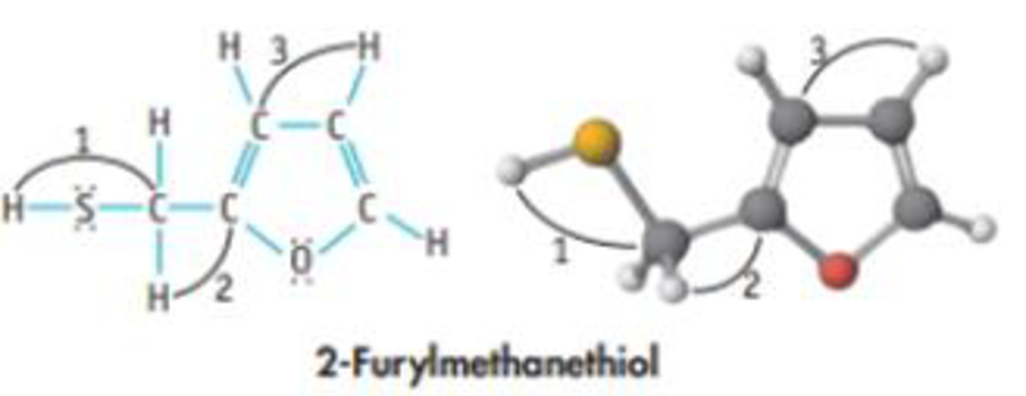# The molecule shown here. 2-furylmelhanethiol, is responsible for the aroma of coffee: (a) What are the formal charges on the S and O atoms? (b) Give approximate values of angles 1, 2, and 3. (c) Which are the shorter carbon-carbon bonds in the molecule? (d) Which bond in this molecule is the most polar? (e) Is the molecule polar or nonpolar? (f) The four C atoms of the ring are all in a plane. Is the O atom in that same plane (making the five-member ring planar), or is the O atom bent above or below the plane?### Chemistry & Chemical Reactivity

9th Edition
John C. Kotz + 3 others
Publisher: Cengage Learning
ISBN: 9781133949640

#### Solutions

Chapter
Section### Chemistry & Chemical Reactivity

9th Edition
John C. Kotz + 3 others
Publisher: Cengage Learning
ISBN: 9781133949640
Chapter 8, Problem 82GQ
Textbook Problem
33 views

## The molecule shown here. 2-furylmelhanethiol, is responsible for the aroma of coffee:(a) What are the formal charges on the S and O atoms? (b) Give approximate values of angles 1, 2, and 3. (c) Which are the shorter carbon-carbon bonds in the molecule? (d) Which bond in this molecule is the most polar? (e) Is the molecule polar or nonpolar? (f) The four C atoms of the ring are all in a plane. Is the O atom in that same plane (making the five-member ring planar), or is the O atom bent above or below the plane?

(a)

Interpretation Introduction

Interpretation: The formal charges on the S and O atoms have to be given.

Concept Introduction:

A formal charge (FC) is the charge assigned to an atom in a molecule, irrespective of relative electronegativity by thinking that electrons in all chemical bonds are shared equally among atoms.

This method is used to identify the most probable Lewis structures if more than one possibility exists for a compound.

The Lewis structure with formal charge on each of the atoms close to zero is taken as the most plausible structure.

Formal charge of an atom can be determined by the given formula.

Formalcharge(FC)=(no.ofvalenceelectroninatom)12(no.ofbondingelectrons)(no.ofnon-bondingelectrons)

### Explanation of Solution

The compound is given below:

The formal charges on the S and O atoms are calculated as:

NumberofvalenceelectronNumberofbondingelectronNumberofnon-bondingelectronFormal </

(b)

Interpretation Introduction

Interpretation: The bond angle in the given compound should be determined.

Concept Introduction:

• In VSEPR, the geometry of the molecule is explained based on minimizing electrostatic repulsion between the molecules’ valence electrons around a central atom
• Bond angle is the angle between two bonds of a molecule and it is determined based on the electron-domain geometry.

[Bond angles: tetrahedral = 109.5o, trigonal planar = 120o, T-shape = 90o]

Electron geometry is the shape of a molecule predicted by considering both bond electron pairs and lone pair of electrons.

Molecular geometry is the shape of a molecule predicted by considering only bond pair of electrons.

Bond angle: It is defined as the angle between the orbital contacting bonding electron pair around the central atom in a molecule ion. Bond angle is expressed in degree.

(c)

Interpretation Introduction

Interpretation:

The shorter carbon–carbon bond in the molecule has to be determined.

Concept introduction:

Bond order: It is the measure of number of electron pairs shared between two atoms.

Bondorder=12(NumberofelectronsinbondoingMOs-NumberofelectronsinantibondingMOs)

Bond length is inversely proportional to the bond order.

(d)

Interpretation Introduction

Interpretation: The most polar bond in the given compound should be determined.

Concept Introduction:

Polarity: It is a well separation of electric charge leading to a molecule or chemical compounds having an electrical dipole moment. Generally the polar molecules must contain polar bonds due to a different in electronegative between the bonded atoms.

(e)

Interpretation Introduction

Interpretation: To find whether the compound is polar or nonpolar.

Concept Introduction:

Electronegativity: It is defined as the capacity of the atom to abstract the pair of electrons towards itself results to have high negative charge.

Polar molecule: The molecule with atoms bonded with different electronegativity. Dipole moment is used to measure the polarity of the molecule.

Polarity of a molecule is measured in term of dipole moment.

Dipole moment for a polar molecule is non-zero and for a non-polar molecule dipole moment is zero.

(f)

Interpretation Introduction

Interpretation: To find whether the oxygen atom in the ring is above or below the plane.

Concept Introduction:

• In VSEPR, the geometry of the molecule is explained based on minimizing electrostatic repulsion between the molecules’ valence electrons around a central atom
• Bond angle is the angle between two bonds of a molecule and it is determined based on the electron-domain geometry.

[Bond angles: tetrahedral = 109.5o, trigonal planar = 120o, T-shape = 90o]

Electron geometry is the shape of a molecule predicted by considering both bond electron pairs and lone pair of electrons.

Molecular geometry is the shape of a molecule predicted by considering only bond pair of electrons.

Bond angle: It is defined as the angle between the orbital contacting bonding electron pair around the central atom in a molecule ion. Bond angle is expressed in degree.

### Still sussing out bartleby?

Check out a sample textbook solution.

See a sample solution

#### The Solution to Your Study Problems

Bartleby provides explanations to thousands of textbook problems written by our experts, many with advanced degrees!

Get Started

Find more solutions based on key concepts
How is temperature related to the density of air?

Fundamentals of Physical Geography

Identify two critical periods of life that demand high nutrient intakes, and defend the use of a vegetarian die...

Nutrition: Concepts and Controversies - Standalone book (MindTap Course List)

Define the following terms: a. chromosome b. chromatin

Human Heredity: Principles and Issues (MindTap Course List)

Come up with a procedure to find the focal length of a concave mirror, such as a spoon.

Physics for Scientists and Engineers: Foundations and Connections

Consider two identical conducting spheres whose surfaces are separated by a small distance. One sphere is given...

Physics for Scientists and Engineers, Technology Update (No access codes included)